Displacement Plot PropertyManager

The Displacement Plot PropertyManager allows you to plot displacement and reaction force results for static, nonlinear, dynamic, drop test studies, or mode shapes for bucking and frequency studies.

To display this PropertyManager, run a static, nonlinear, dynamic study, or drop test study. Right-click Results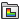and select Define Displacement Plot.

Displacement results are available for nodes only.

Display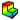Component Select a displacement or reaction force component to plot. Directions are based on the selected reference geometry. See Displacement and Reaction Force Components.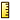Units Select a unit for the displacement plot. You can control the display of the fringe plot using the Settings PropertyManager.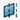Plane, Axis or Coordinate System Select a reference plane or a reference axis to plot displacement. Show PSD value Available only for random vibration studies. When checked, the PSD values are shown for a particular solution step. Otherwise, the RMS values across the whole frequency range are shown. Show as vector plot Generates a vector plot where a vector is plotted at each node to show the magnitude and direction of the selected component. You can control the size and density of the vectors in a vector plot using the Vector Plot Options PropertyManager. Available for directional displacement components only. Render the thickness for shells (slower) Displays the displacement results on shell bodies using a 3D representation of the shells. For each node, one value is shown across the shell thickness. The shell body is displayed with the thickness value defined in the Shell Definition PropertyManager. The shell thickness orientation is displayed with relation to the midsurface of the shell as defined by the offset value in the Shell Definition PropertyManager. Show plot only on selected entities Generates plots only for the selected entities.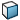Select faces for the plot Select the faces to view results.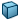Select bodies for the plot Select the bodies to view results. Display symmetric results Available for models with symmetry restraints or cyclic symmetry. Select to view the displacement values for the whole model. The results are mirrored about the planes of symmetry for models where you analyze half, a quarter, or one-eighth of the actual geometry. For cyclic symmetry, results are repeated cyclically about the defined axis of revolution. Render beam profile (slower) Available only for studies with beams. Displays displacement plot on actual beam geometry as opposed to cylinders.

Plot Step

Available only for nonlinear, dynamic and drop test studies.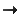Plot for Single Step Select this option to plot the results at the selected solution step.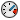Sets the time (in seconds) at which the displacement results are plotted. If there are no results available for the requested time, results are plotted for the nearest available solution step number.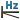Available for harmonic and random vibration studies. Displays the frequency corresponding to the selected plot step.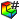Plot Step Sets the solution step number for the plot.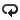Plot Bounds across All Steps Select this option to plot extreme values regardless of the solution step they occur at. Select one of the following:
 Maximum Displays the maximum algebraic values (envelope plot) across all solution steps. Minimum Displays the minimum algebraic values (envelope plot) across all solution steps. Absolute maximum Available only for frequency and buckling studies. Displays the absolute maximum values (envelope plot) across all solution steps.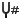Mode Shape Sets the mode shape number for which the displacement is to be plotted.Frequency Displays the frequency corresponding to the specified mode shape.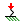Load Factor Displays the buckling load factor.

Deformed Shape

If checked, the selected component is plotted on the deformed shape of the model.

 Automatic Displays the default Scale Factor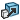that the program uses to scale the largest deformation to 10% of the largest dimension of the smallest box that surrounds the model. True scale Displays the actual deformed shape of the model (Scale Factor is 1.0). User defined Allows you to enter your own Scale Factor. In most cases, a larger scale factor helps to appreciate the deflection. Show colors Toggles the visibility of the displacement plot on the deformed shape of the model.

Property

 Include title text Type a custom title for the plot. Associate plot with name view orientation Associate a predefined view orientation with the active plot.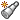Associate with current view Associates the current view with the active plot.